mscroggs.co.uk
mscroggs.co.uksubscribe

# Blog

2019-06-19
Last night at MathsJam, Peter Kagey showed me a conjecture about OEIS sequence A308092.A308092
The sum of the first $$n$$ terms of the sequence is the concatenation of the first $$n$$ bits of the sequence read as binary, with $$a(1) = 1$$.
1, 2, 3, 7, 14, 28, 56, 112, 224, 448, 896, 1791, 3583, 7166, ...To understand this definition, let's look at the first few terms of this sequence written in binary:
1, 10, 11, 111, 1110, 11100, 111000, 1110000, 11100000, 111000000, ...
By "the concatenation of the first $$n$$ bits of the sequence", it means the first $$n$$ binary digits of the whole sequence written in order: 1, then 11, then 110, then 1101, then 11011, then 110111, and so on. So the definition means:
• The first term is 1, as given in the definition ($$a(1)=1$$).
• The sum of the first 2 terms is the first 2 digits: $$1+10=11$$.
• The sum of the first 3 terms is the first 3 digits: $$1+10+11=110$$.
• The sum of the first 4 terms is the first 4 digits: $$1+10+11+111=1101$$.
• The sum of the first 5 terms is the first 5 digits: $$1+10+11+111+1110=11011$$.
As we know that the sum of the first $$n-1$$ terms is the first $$n-1$$ digits, we can calculate the third term of this sequence onwards using: "$$a(n)$$ is the concatenation of the first $$n$$ bits of the sequence subtract concatenation of the first $$n-1$$ bits of the sequence":
• The third term is $$110 - 11 = 11$$.
• The fourth term is $$1101 - 110 = 111$$.
• The fourth term is $$11011 - 1101 = 1110$$.
• The fifth term is $$110111 - 11011 = 11100$$.

### The conjecture

Peter's conjecture is that the number of 1s in each term is greater than or equal to the number of 1s in the previous term.
I'm going to prove this conjecture. If you'd like to have a try first, stop reading now and come back when you're ready for spoilers. (If you'd like a hint, read the next section then pause again.)

The third term of the sequence onwards can be calculated by subtracting the first $$n-1$$ digits from the first $$n$$ digits. If the first $$n-1$$ digits form a binary number $$x$$, then the first $$n$$ digits will be $$2x+d$$, where $$d$$ is the $$n$$th digit (because moving all the digits to the left one place in binary is multiplying by two).
Therefore the different is $$2x+d-x=x+d$$, and so we can work out the $$n$$th term of the sequence by adding the $$n$$th digit in the sequence to the first $$n-1$$ digits. (Hat tip to Martin Harris, who spotted this first.)

### Carrying

Adding 1 to a binary number the ends in 1 will cause 1 to carry over to the left. This carrying will continue until the 1 is carried into a position containing 0, and after this all the digits to the left of this 0 will remain unchanged.
Therefore adding a digit to the first $$n-1$$ digits can only change the digits from the rightmost 0 onwards.

### Endings

We can therefore disregard all the digits before the rightmost 0, and look at how the $$n$$th term compares to the $$(n-1)$$th term. There are 5 ways in which the first $$n$$ digits could end:
• $$00$$
• $$010$$
• $$01...10$$ (where $$1...1$$ is a string of 2 or more ones)
• $$01$$
• $$01...1$$ (where $$1...1$$ is again a string of 2 or more ones)
We now look at each of these in turn and show that the $$n$$th term will contain at least as many ones at the $$(n-1)$$th term.

#### Case 1: $$00$$

If the first $$n$$ digits of the sequence are $$x00$$ (a binary number $$x$$ followed by two zeros), then the $$(n-1)$$th term of the sequence is $$x+0=x$$, and the $$n$$th term of the sequence is $$x0+0=x0$$. Both $$x$$ and $$x0$$ contain the same number of ones.

#### Case 2: $$010$$

If the first $$n$$ digits of the sequence are $$x010$$, then the $$(n-1)$$th term of the sequence is $$x0+1=x1$$, and the $$n$$th term of the sequence is $$x01+0=x01$$. Both $$x1$$ and $$x01$$ contain the same number of ones.

#### Case 3: $$01...10$$

If the first $$n$$ digits of the sequence are $$x01...10$$, then the $$(n-1)$$th term of the sequence is $$x01...1+1=x10...0$$, and the $$n$$th term of the sequence is $$x01...10+1=x01...1$$. $$x01...1$$ contains more ones than $$x10...0$$.

#### Case 4: $$01$$

If the first $$n$$ digits of the sequence are $$x01$$, then the $$(n-1)$$th term of the sequence is $$x+0=x$$, and the $$n$$th term of the sequence is $$x0+1=x1$$. $$x1$$ contains one more one than $$x$$.

#### Case 5: $$01...1$$

If the first $$n$$ digits of the sequence are $$x01...1$$, then the $$(n-1)$$th term of the sequence is $$x01...1+1=x10...0$$, and the $$n$$th term of the sequence is $$x01...1+1=x10...0$$. Both these contain the same number of ones.

In all five cases, the $$n$$th term contains more ones or an equal number of ones to the $$(n-1)$$th term, and so the conjecture is true.

### Similar posts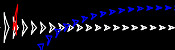Mathsteroids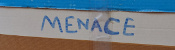Building MENACEs for other gamesBig Ben Strikes AgainChristmas (2016) is over

Comments in green were written by me. Comments in blue were not written by me.

Allowed HTML tags: <br> <a> <small> <b> <i> <s> <sup> <sub> <u> <spoiler> <ul> <ol> <li>
To prove you are not a spam bot, please type "nogaxeh" backwards in the box below (case sensitive):
2018-07-06
This is a post I wrote for round 1 of The Aperiodical's Big Internet Math-Off 2018, where Mathsteroids lost to MENACE.
A map projection is a way of representing the surface of a sphere, such as the Earth, on a flat surface. There is no way to represent all the features of a sphere on a flat surface, so if you want a map that shows a certain feature of the world, then you map will have to lose some other feature of the world in return.
To show you what different map projections do to a sphere, I have created a version of the game Asteroids on a sphere. I call it Mathsteroids. You can play it here, or follow the links below to play on specific projections.

### Mercator projection

The most commonly used map projection is the Mercator projection, invented by Gerardus Mercator in 1569. The Mercator projection preserves angles: if two straight lines meet at an angle $$\theta$$ on a sphere, then they will also meet at an angle $$\theta$$ on the map. Keeping the angles the same, however, will cause the straight lines to no longer appear straight on the map, and the size of the same object in two different place to be very different.
The angle preserving property means that lines on a constant bearing (eg 030° from North) will appear straight on the map. These constant bearing lines are not actually straight lines on the sphere, but when your ship is already being buffeted about by the wind, the waves, and the whims of drunken sailors, a reasonably straight line is the best you can expect.
The picture below shows what three ships travelling in straight lines on the sphere look like on a Mercator projection.
To fully experience the Mercator projection, you can play Mathsteroids in Mercator projection mode here. Try flying North to see your spaceship become huge and distorted.

### Gall–Peters projection

The Gall–Peters projection (named after James Gall and Arno Peters) is an area-preserving projection: objects on the map will have the same area as objects on the sphere, although the shape of the object on the projection can be very different to its shape on the sphere.
The picture below shows what three spaceships travelling in straight lines on a sphere look like on the Gall–Peters projection.
You can play Mathsteroids in Gall–Peters projection mode here. I find this one much harder to play than the Mercator projection, as the direction you're travelling changes in a strange way as you move.

### Azimuthal projection

An azimuthal projection makes a map on which the directions from the centre point to other points on the map are the same as the directions on the sphere. A map like this could be useful if, for example, you're a radio operator and need to quickly see which direction you should point your aerial to communicate with other points on the map.
The azimuthal projection I've used in Mathsteroids also preserves distances: the distance from the centre to the another points on the map is proportional to the actual distance on the sphere. This projection is used as the emblem of the UN.
The picture below shows three straight lines on this projection. You can play Mathsteroids in azimuthal mode here.### Craig retroazimuthal projection

A retroazimuthal projection makes a map on which the directions to the centre point from other points on the map are the same as the directions on the sphere. If you're thinking that this is the same as the azimuthal projection, then you're too used to doing geometry on flat surfaces: on a sphere, the sum of the angles in a triangle depends on the size of the triangle, so the directions from A to B and from B to A aren't as closely related as you would expect.
The Craig retroazimuthal projection was invented by James Ireland Craig in 1909. He used Mecca as his centre point to make a map that shows muslims across the world which direction they should face to pray.
The picture below shows what three spaceships travelling in a straight lines on a sphere looks like on this projection.
You can play Mathsteroids in Craig retroazimuthal mode here to explore the projection yourself. This is perhaps the hardest of all to play, as (a) two different parts of the sphere overlap on the map, and (b) the map is actually infinitely tall, so quite a bit of it is off the edge of the visible game area.

### Stereographic projection

The final projection I'd like to show you is the stereographic projection.
Imagine that a sphere is sitting on a 2D plane. Take a point on the sphere. Imagine a straight line through this point and the point at the top of the sphere. The point where this line meets the 2D plane is stereographic projection of the point on the sphere.
This projection (backwards) can be used to represent the every complex number as a point on a sphere: this is called the Riemann sphere.
To make Mathseteroids playable after this projection, I split the sphere into 2 hemisphere and projected each seperately to give two circles. You can play Mathsteroids in stereographic projection mode here. Three spaceships travelling in straight lines on this projection are shown below.
... and if you still don't like map projections, you can still enjoy playing Mathsteroids on an old fashioned torus. Or on a Klein bottle or the real projective plane. Don't forget to take a short break from playing to head over to The Aperiodical and vote (voting now closed).

### Similar posts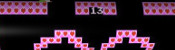Video game surfacesBig Internet Math-Off stickersRunge's PhenomenonBuilding MENACEs for other games

Comments in green were written by me. Comments in blue were not written by me.

Allowed HTML tags: <br> <a> <small> <b> <i> <s> <sup> <sub> <u> <spoiler> <ul> <ol> <li>
To prove you are not a spam bot, please type "elbatnuocnu" backwards in the box below (case sensitive):
2018-04-29
Two years ago, I built a copy of MENACE (Machine Educable Noughts And Crosses Engine). Since then, it's been to many Royal Institution masterclasses, visted Manchester and met David Attenborough. When I'm not showing them off, the 304 matchboxes that make up my copy of MENACE live in this box:
This box isn't very big, which might lead you to wonder how big MENACE-style machines would be for other games.

### Hexapawn (HER)

In A matchbox game learning-machine by Martin Gardner , the game of Hexapawn was introduced. Hexapawn is played on a 3×3 grid, and starts with three pawns facing three pawns.
The pieces move like pawns: they may be either moved one square forwards into an empty square, or take another pawn diagonally (the pawns are not allowed to move forwards two spaces on their first move, as they can in chess). You win if one of your pawns reaches the other end of the board. You lose if none of your pieces can move.
The game was invented by Martin Gardner as a good game for his readers to build a MENACE-like machine to play against, as there are only 19 positions that can face player two, so only 19 matchboxes are needed to make HER (Hexapawn Educable Robot). (HER plays as player two, as if player two plays well they can always win.)

### Nine Men's Morris (MEME)

In Nine Men's Morris, two players first take turns to place pieces on the board, before taking turns to move pieces to adjacent spaces. If three pieces are placed in a row, a player may remove one of the opponent's pieces. It's a bit like Noughts and Crosses, but with a bit more chance of it not ending in a draw.
In Solving Nine Men's Morris by Ralph Gasser , the number of possible game states in Nine Men's Morris is given as approximately $$10^{10}$$. To build MEME (Machine Educable Morris Engine), you would need this many matchboxes. These boxes would form a sphere with radius 41m: that's approximately the length of two tennis courts.
As a nice bonus, if you build MEME, you'll also smash the world record for the largest matchbox collection.

### Connect 4 (COFFIN)

In Symbolic classification of general two-player games by Stefan Edelkamp and Peter Kissmann , the number of possible game states in Connect 4 is given as 4,531,985,219,092. The boxes used to make COFFIN (COnnect Four Fighting INstrument) would make a sphere with radius 302m: approximately the height of the Eiffel Tower.COFFIN: COnnect Four Fighting INstrument

### Draughts/Checkers (DOCILE)

In Solving the game of Checkers by Jonathan Schaeffer and Robert Lake , the number of possible game states in Draughts is given as approximately $$5\times10^{20}$$. The boxes needed to build DOCILE (Draughts Or Checkers Intelligent Learning Engine) would make a sphere with radius 151km.
DOCILE: Draughts Or Checkers Intelligent Learning Engine
If the centre of DOCILE was in London, some of the boxes would be in Sheffield.

### Chess (CLAWS)

The number of possible board positions in chess is estimated to be around $$10^{43}$$. The matchboxes needed to make up CLAWS (Chess Learning And Winning System) would fill a sphere with radius $$4\times10^{12}$$m.
If the Sun was at the centre of CLAWS, you might have to travel past Uranus on your search for the right box.

### Go (MEGA)

The number of possible positions in Go is estimated to be somewhere near $$10^{170}$$. To build MEGA (Machine Educable Go Appliance), you're going to need enough matchboxes to make a sphere with radius $$8\times10^{54}$$m.
The observable universe takes up a tiny space at the centre of this sphere. In fact you could fit around $$10^{27}$$ copies of the universe side by side along the radius of this sphere.
It's going to take you a long time to look through all those matchboxes to find the right one...

#### References

A matchbox game learning-machine by Martin Gardner. Scientific American, March 1962. [link]
Solving Nine Men's Morris by Ralph Gasser. Games of No Chance 29, 1996. [link]
Symbolic classification of general two-player games by Stefan Edelkamp and Peter Kissmann. in Advances in Artificial Intelligence (edited by A.R. Dengel, K. Berns, T.M. Breuel, F. Bomarius, T.R. Roth-Berghofer), 2008. [link]
Solving the game of Checkers by Jonathan Schaeffer and Robert Lake. Games of No Chance 29, 1996. [link]

### Similar posts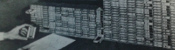MENACEMENACE in fiction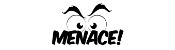MENACE at Manchester Science Festival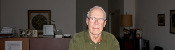The Mathematical Games of Martin Gardner

Comments in green were written by me. Comments in blue were not written by me.
2018-11-16
Of course, to make CLAWS, you will have to leave a large gap in the centre of the matchbox sphere, to avoid the very real danger of fire. Furthermore, some redundancy is needed, to replace the boxes which will be damaged by the myriad hard objects which are whizzing around the solar system. For this latter reason alone, I propose that the machine would be impractical to make! ;-D

Allowed HTML tags: <br> <a> <small> <b> <i> <s> <sup> <sub> <u> <spoiler> <ul> <ol> <li>
To prove you are not a spam bot, please type "tneitouq" backwards in the box below (case sensitive):
2017-06-03
As a child, I was a huge fan of Captain Scarlet and the Mysterons, Gerry Anderson's puppet-starring sci-fi series. Set in 2068, the series follows Captain Scarlet and the other members of Spectrum as they attempt to protect Earth from the Mysterons. One of my favourite episodes of the series is the third: Big Ben Strikes Again.
In this episode, the Mysterons threaten to destroy London. They do this by hijacking a vehicle carrying a nuclear device, and driving it to a car park. In the car park, the driver of the vehicle wakes up and turns the radio on. Then something weird happens: Big Ben strikes thirteen!The driver turning on the radio. Good to know that BBC Radio 4 will still broadcast at 92-95FM in 2068.
Following this, the driver is knocked out again and wakes up in a side road somewhere. After hearing his story, Captain Blue works out that the car park must be 1500 yards away from Big Ben. Using this information, Captains Blue and Scarlet manage to track down the nuclear device and save the day.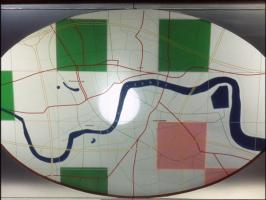A map of London with a circle of radius 1500 yards drawn on it.
After rewatching the episode recently, I realised that it would be possible to recreate this scene and hear Big Ben striking thirteen.

### Where does Big Ben strike thirteen?

At the end of the episode, Captain Blue explains to Captain Scarlet that the effect was due to light travelling faster than sound: as the driver had the radio on, he could hear Ben's bongs both from the tower and through the radio. As radio waves travel faster than sound, the bongs over the radio can be heard earlier than the sound waves travelling through the air. Further from the tower, the gap between when the two bongs are heard is longer; and at just the right distance, the second bong on the radio will be heard at the same time as the first bong from the tower. This leads to the appearance of thirteen bongs: the first bong is just from the radio, the next eleven are both radio and from the tower, and the final bong is only from the tower.
Big Ben's bongs are approximately 4.2s apart, sound travels at 343m/s, and light travels at 3×108m/s (this is so fast that it could be assumed that the radio waves arrive instantly without changing the answer). Using these, we perform the following calculation:
$$\text{time difference} = \text{time for sound to arrive}-\text{time for light to arrive}$$ $$=\frac{\text{distance}}{\text{speed of sound}}-\frac{\text{distance}}{\text{speed of light}}$$ $$=\text{distance}\times\left(\frac1{\text{speed of sound}}-\frac1{\text{speed of light}}\right)$$ $$\text{distance}=\text{time difference}\div\left(\frac1{\text{speed of sound}}-\frac1{\text{speed of light}}\right)$$ $$=4.2\div\left(\frac1{343}-\frac1{3\times10^8}\right)$$ $$=1440\text{m}\text{ or }1574\text{ yards}$$
This is close to Captain Blue's calculation of 1500 yards (and to be fair to the Captain, he had to calculate it in his head in a few seconds). Plotting a circle of this radius centred at Big Ben gives the points where it may be possible to hear 13 bongs.
Again, the makers of Captain Scarlet got this right: their circle shown earlier is a very similar size to this one. To demonstrate that this does work (and with a little help from TD and her camera), I made the following video yesterday near Vauxhall station. I recommend using earphones to watch it as the later bongs are quite faint.

### Similar posts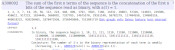Proving a conjectureMathsteroidsBuilding MENACEs for other gamesTube map kaleidocycles

Comments in green were written by me. Comments in blue were not written by me.
2018-11-16
@g0mrb: Thanks for letting me know, I'll look into it...
2018-11-15
There is no sound in this video, using Safari in iOS 12.1.1 Beta.
2017-06-04
This is awesome and wonderful. I salute you.
2017-06-03
Wow! This has made my weekend.

Allowed HTML tags: <br> <a> <small> <b> <i> <s> <sup> <sub> <u> <spoiler> <ul> <ol> <li>
To prove you are not a spam bot, please type "width" in the box below (case sensitive):
2015-08-27
In 1961, Donald Michie build MENACE (Machine Educable Noughts And Crosses Engine), a machine capable of learning to be a better player of Noughts and Crosses (or Tic-Tac-Toe if you're American). As computers were less widely available at the time, MENACE was built from from 304 matchboxes.Taken from Trial and error by Donald Michie 
The original MENACE.
To save you from the long task of building a copy of MENACE, I have written a JavaScript version of MENACE, which you can play against here.

### How to play against MENACE

To reduce the number of matchboxes required to build it, MENACE aways plays first. Each possible game position which MENACE could face is drawn on a matchbox. A range of coloured beads are placed in each box. Each colour corresponds to a possible move which MENACE could make from that position.
To make a move using MENACE, the box with the current board position must be found. The operator then shakes the box and opens it. MENACE plays in the position corresponding to the colour of the bead at the front of the box.
For example, in this game, the first matchbox is opened to reveal a red bead at its front. This means that MENACE (O) plays in the corner. The human player (X) then plays in the centre. To make its next move, MENACE's operator finds the matchbox with the current position on, then opens it. This time it gives a blue bead which means MENACE plays in the bottom middle.
The human player then plays bottom right. Again MENACE's operator finds the box for the current position, it gives an orange bead and MENACE plays in the left middle. Finally the human player wins by playing top right.
MENACE has been beaten, but all is not lost. MENACE can now learn from its mistakes to stop the happening again.

### How MENACE learns

MENACE lost the game above, so the beads that were chosen are removed from the boxes. This means that MENACE will be less likely to pick the same colours again and has learned. If MENACE had won, three beads of the chosen colour would have been added to each box, encouraging MENACE to do the same again. If a game is a draw, one bead is added to each box.
Initially, MENACE begins with four beads of each colour in the first move box, three in the third move boxes, two in the fifth move boxes and one in the final move boxes. Removing one bead from each box on losing means that later moves are more heavily discouraged. This helps MENACE learn more quickly, as the later moves are more likely to have led to the loss.
After a few games have been played, it is possible that some boxes may end up empty. If one of these boxes is to be used, then MENACE resigns. When playing against skilled players, it is possible that the first move box runs out of beads. In this case, MENACE should be reset with more beads in the earlier boxes to give it more time to learn before it starts resigning.

### How MENACE performs

In Donald Michie's original tournament against MENACE, which lasted 220 games and 16 hours, MENACE drew consistently after 20 games.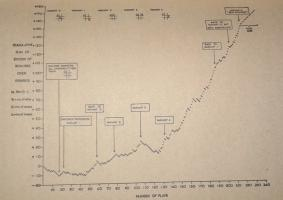Taken from Trial and error by Donald Michie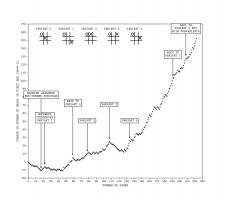Graph showing MENACE's performance in the original tournament. Edit: Added the redrawn graph on the left.
After a while, Michie tried playing some more unusual games. For a while he was able to defeat MENACE, but MENACE quickly learnt to stop losing. You can read more about the original MENACE in A matchbox game learning-machine by Martin Gardner  and Trial and error by Donald Michie .
You may like to experiment with different tactics against MENACE yourself.

### Play against MENACE

I have written a JavaScript implemenation of MENACE for you to play against. The source code for this implementation is available on GitHub.
When playing this version of MENACE, the contents of the matchboxes are shown on the right hand side of the page. The numbers shown on the boxes show how many beads corresponding to that move remain in the box. The red numbers show which beads have been picked in the current game.
The initial numbers of beads in the boxes and the incentives can be adjusted by clicking Adjust MENACE's settings above the matchboxes. My version of MENACE starts with more beads in each box than the original MENACE to prevent the early boxes from running out of beads, causing MENACE to resign.
Additionally, next to the board, you can set MENACE to play against random, or a player 2 version of MENACE.
Edit: After hearing me do a lightning talk about MENACE at CCC, Oliver Child built a copy of MENACE. Here are some pictures he sent me: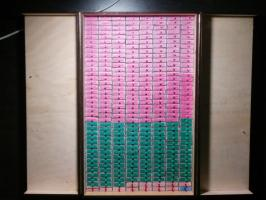Edit: Oliver has written about MENACE and the version he built in issue 03 of Chalkdust Magazine.
Edit: Inspired by Oliver, I have built my own MENACE. I took it to the MathsJam Conference 2016. It looks like this: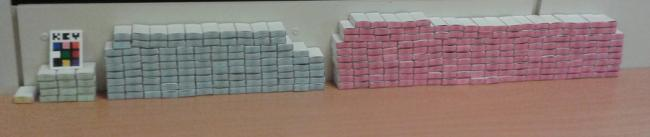#### References

A matchbox game learning-machine by Martin Gardner. Scientific American, March 1962. [link]
Trial and error by Donald Michie. Penguin Science Survey, 1961.

### Similar postsBuilding MENACEs for other gamesMENACE at Manchester Science FestivalMENACE in fictionThe Mathematical Games of Martin Gardner

Comments in green were written by me. Comments in blue were not written by me.
2019-07-06
Can you make a version where the ai goes second?
2019-04-09
@Sterling: To make a MENACE to play chess, you'd need to draw every possible position in chess. As I wrote in this blog post, if you had a matchbox for each possible chess position, your pile of matchboxes would be this big: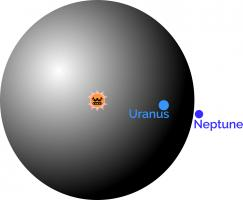This number of positions is too big for this method of learning to work for chess, so you'd need a more advanced method...
2019-04-08
After watching the video with yourself and Matt, you commented on teaching it chess are there any PDFs on how to teach, the machine chess. Ie what are the mechanics
2019-03-27
@Alex: You can find the pdfs here. boxes0.pdf to boxes3.pdf are the states for a MENACE that goes first. second_boxes0.pdf to second_boxes2.pdf are the states for a MENACE that goes second.
2019-03-26
I know this has been asked a lot but would it be possible if you could make a pdf of all possible states of the game on your website? If not please just send them to me directly. Thank you, alex